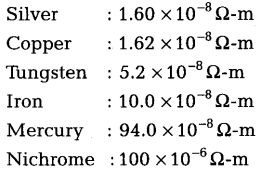# The potential difference between two points in an electric circuit is 1 V

The potential difference between two points in an electric circuit is 1 V What does it mean? Name a device that helps to measure the potential difference across a conductor.
(ii) Why does the connecting cord of an - electric heater not glow while the heating element does?
(iii) Electrical resistivities of some substances at 20 °C are given as below:(a) Among silver and copper, which one is a better conductor and why?
(b) Which material would you advise to be used in electrical heating devices and why?

(i) The potential difference between two points is 1 V, means that if a charge of 1 C is moved from one point to the other, then 1 J of work is required.The potential difference across a conductor is measured by means of an instrument called the voltmeter.
(ii) The electric power ft is given by [P = ;{I^2}R]
The resistance of the heating element is very high. Large amount of heat generates in the heating element and it glows hot.
The resistance of connecting cord is very low. Thus, negligible heat generates in the connecting cord and it does not glow.
(iii)
(a) Silver is a better conductor due to its lower resistivity.
(b) Nichrome should be used in electrical heating devices due to very high resistivity.